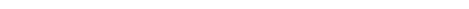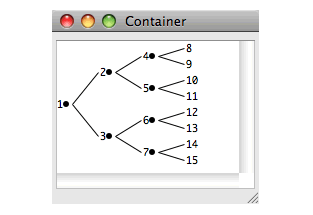### 5.6 Graph panes

Another kind of choice is the graph-pane. This is a special pane that can draw graphs, whose nodes and edges can be selected, and for which callbacks can be specified, as usual.

While graph-pane is a subclass of choice and hence collection, the concept of collection items is not applicable to a graph. Instead, the items in a graph-pane are constructed from a list of "roots" (arbitrary objects) which are specified by the initarg `:roots` and can be accessed later by `graph-pane-roots`, and a children-function . The roots define the initial nodes, and when the user expands a node, the children-function is called to compute the children, which is a list of more items, which specify the children nodes of the expanded node. Thus the actual items in the graph are changed as nodes are expanded or collapsed.

The concepts of selection, that is the functions choice-selected-items and so on, are applicable to graph-pane.

Here is a simple example of a graph pane. It draws a small rooted tree:

`(contain `
` (make-instance`
`  'graph-pane`
`  :roots '(1)`
`  :children-function `
`  #'(lambda (x)`
`      (when (< x 8)`
`       (list (* 2 x) (1+ (* 2 x))))))) `

Figure 5.7 A graph paneThe graph pane is supplied with a `:children-function` which it uses to calculate the children of the root node, and from those children it continues to calculate more children until the termination condition is reached. For more details of this, see the manual page for graph-pane.

graph-pane provides a gesture which expands or collapses a node, depending on its current state. Click on the circle alongside the node to expand or collapse it.

You can associate selection, retraction, extension, and action callbacks with any or all elements of a graph. Here is a simple graph pane that has an action callback on its nodes.

First we need a pane which will display the callback messages. Executing the following form to create this pane:

`(defvar *the-collector*`
`   (contain (make-instance 'collector-pane)))`

Then, define the following four callback functions:

`(defun test-action-callback (&rest args)`
`            (format (collector-pane-stream `
`                     *the-collector*) "Action"))`
`(defun test-selection-callback (&rest args)`
`            (format (collector-pane-stream *the-collector*)`
`                    "Selection"))`
`(defun test-extend-callback (&rest args)`
`            (format (collector-pane-stream *the-collector*)`
`                    "Extend"))`
`(defun test-retract-callback (&rest args)`
`            (format (collector-pane-stream *the-collector*)`
`                    "Retract"))`

Now create an extended selection graph pane which uses each of these callbacks, the callback used depending on the action taken:

`(contain`
`   (make-instance`
`      'graph-pane `
`      :interaction :extended-selection`
`      :roots '(1)`
`      :children-function`
`       #'(lambda (x)`
`            (when (< x 8)`
`                  (list (* 2 x) (1+ (* 2 x)))))`
`      :action-callback 'test-action-callback`
`      :selection-callback 'test-selection-callback`
`      :extend-callback 'test-extend-callback`
`      :retract-callback 'test-retract-callback))`

The selection callback function is called whenever any node in the graph is selected.

The extension callback function is called when the selection is extended by middle clicking on another node (thus selecting it too).

The retract callback function is called whenever an already selected node is deselected.

The action callback function is called whenever an action is performed on a node (that is, whenever it gets a double-click, or `Return` is pressed while the node is selected).

#### 5.6.1 Changing the graphics in the graph

graph-pane is actually a subclass of pinboard-layout, and displays the graph using elements (normally pinboard-object, but can also be simple-pane). You can specify the class of these elements, as well as a function to actually create the object for each node. This allows you to modify the appearance of the graph without affecting or accessing the topology of the graph.

You can also access the element that displays a graph-object by the reader `graph-object-element`, and manipulate it directly. See for example:

``` (example-edit-file "capi/graphics/graph-color-edges.lisp")```

#### 5.6.2 Controlling the layout

The roots of the graph are placed at one side of the panes and the graph grows into the pane. The side on which the roots are placed is defined by the layout-function and accessor `graph-pane-layout-function`, which takes one of the keyword values `:left-right`, `:top-down`, `:right-left` and `:bottom-up`, where the first word in a keyword is the side where the roots are placed. There is also an accessor `graph-pane-direction`, which maps `:forward` to/from `:left-right` and `:left-right`, and maps `:backward` to/from `:right-left` and `:bottom-up`, which makes it easier to set the direction without changing the vertical/horizontal dimension.

#### 5.6.3 Accessing the topology of the graph

The topology of the graph is represented by graph-node objects and graph-edge objects. The list of graph-nodes and graph-edges of the graph-pane can be found by graph-pane-edges and graph-pane-nodes. Note, however, that these are subject to change as the user interacts with the graph.

You can find the node associated with an item (if any) by using find-graph-node. You can find the children of a supplied node by graph-node-children. You can find the edges from the node (that is, to its children) by the reader `graph-node-out-edges`, and edges in by `graph-node-in-edges`. You can also search for an edge between a parent and child by find-graph-edge. From a graph-edge, you can find the the parent and child that are connected by it by the accessors `graph-edge-from` and `graph-edge-to` respectively. It is possible to select specific nodes by graph-pane-select-graph-nodes, which takes a predicate that is applied to all the nodes.

You can find the geometry of a node, that is the part of the pane occupied by the element that is associated with the node, by the graph-node readers `graph-node-x`, `graph-node-y`, `graph-node-height` and `graph-node-width`. You can find whether a point in the pane is within the area of a graph object, either a graph-node or graph-edge, by using graph-pane-object-at-position.

It is possible to modify the graph explicitly by graph-pane-delete-object, graph-pane-delete-objects, graph-pane-delete-selected-objects and graph-pane-add-graph-node. However, that will be overridden next time the graph-pane computes the layout.

The user can interactively move nodes (and hence also edges) in the graph. If you need to know when that happens, you make a subclass of graph-pane, and then specialize graph-pane-update-moved-objects on it.

graph-node and graph-edge are both subclasses of graph-object, and inherit from it the readers `graph-object-object`, which returns the graph item associated with the graph-object, and `graph-object-element`, which returns the element that displays it (normally pinboard-object, but can also be simple-pane).

CAPI User Guide and Reference Manual (Macintosh version) - 3 Aug 2017